Courses

# Test: CPU & I/O Scheduling- 3

## 15 Questions MCQ Test Operating System | Test: CPU & I/O Scheduling- 3

Description
This mock test of Test: CPU & I/O Scheduling- 3 for Computer Science Engineering (CSE) helps you for every Computer Science Engineering (CSE) entrance exam. This contains 15 Multiple Choice Questions for Computer Science Engineering (CSE) Test: CPU & I/O Scheduling- 3 (mcq) to study with solutions a complete question bank. The solved questions answers in this Test: CPU & I/O Scheduling- 3 quiz give you a good mix of easy questions and tough questions. Computer Science Engineering (CSE) students definitely take this Test: CPU & I/O Scheduling- 3 exercise for a better result in the exam. You can find other Test: CPU & I/O Scheduling- 3 extra questions, long questions & short questions for Computer Science Engineering (CSE) on EduRev as well by searching above.
QUESTION: 1

### Consider the following snapshot of a system running n processes. Process i is holding Xi instances of a resource R, 1 <= i <= n. currently, all instances of R are occupied. Further, for all i, process i has placed a request for an additional Yi instances while holding the Xi instances it already has. There are exactly two processes p and q such that Yp = Yq = 0. Which one of the following can serve as a necessary condition to guarantee that the system is not approaching a deadlock?

Solution:

Since both p and q don’t need additional resources, they both can finish and release Xp + Xq resources without asking for any additional resource. If the resources released by p and q are sufficient for another process waiting for Yk resources, then system is not approaching deadlock.

QUESTION: 2

### Suppose n processes, P1, …. Pn share m identical resource units, which can be reserved and released one at a time. The maximum resource requirement of process Pi is Si, where Si > 0. Which one of the following is a sufficient condition for ensuring that deadlock does not occur?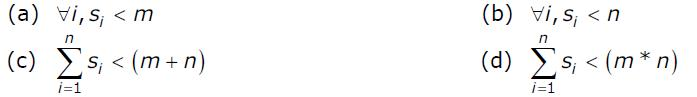Solution:

In the extreme condition, all processes acquire Si-1 resources and need 1 more resource. So following condition must be true to make sure that deadlock never occurs.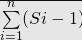< m The above expression can be written as following.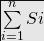< (m + n)

QUESTION: 3

### A system has n resources R0,...,Rn-1,and k processes P0,....Pk-1.The implementation of the resource request logic of each process Pi is as follows:  if (i % 2 == 0) {       if (i < n) request Ri       if (i+2 < n) request Ri+2 } else {       if (i < n) request Rn-i       if (i+2 < n) request Rn-i-2 } In which one of the following situations is a deadlock possible?

Solution:

No. of resources, n = 21
No. of processes, k = 12

Processes {P0, P1....P11}  make the following Resource requests:
{R0, R20, R2, R18, R4, R16, R6, R14, R8, R12, R10, R10}

For example P0 will request R0 (0%2 is = 0 and 0< n=21).

Similarly, P10 will request R10.

P11 will request R10 as n - i = 21 - 11 = 10.

As different processes are requesting the same resource, deadlock
may occur.

QUESTION: 4

Three concurrent processes X, Y, and Z execute three different code segments that access and update certain shared variables. Process X executes the P operation (i.e., wait) on semaphores a, b and c; process Y executes the P operation on semaphores b, c and d; process Z executes the P operation on semaphores c, d, and a before entering the respective code segments. After completing the execution of its code segment, each process invokes the V operation (i.e., signal) on its three semaphores. All semaphores are binary semaphores initialized to one. Which one of the following represents a deadlockfree order of invoking the P operations by the processes?

Solution:

Option A can cause deadlock. Imagine a situation process X has acquired a, process Y has acquired b and process Z has acquired c and d. There is circular wait now. Option C can also cause deadlock. Imagine a situation process X has acquired b, process Y has acquired c and process Z has acquired a. There is circular wait now. Option D can also cause deadlock. Imagine a situation process X has acquired a and b, process Y has acquired c. X and Y circularly waiting for each other.

Consider option A) for example here all 3 processes are concurrent so X will get semaphore a, Y will get b and Z will get c, now X is blocked for b, Y is blocked for c, Z gets d and blocked for a. Thus it will lead to deadlock. Similarly one can figure out that for B) completion order is Z,X then Y.

QUESTION: 5

Which of the following is NOT true of deadlock prevention and deadlock avoidance schemes?

Solution:

Deadlock Prevention: Deadlocks can be prevented by preventing at least one of the four required conditions:

1. Mutual Exclusion – not required for sharable resources; must hold for non-sharable resources.

2. Hold and Wait – must guarantee that whenever a process requests a resource, it does not hold any other resources. Require process to request and be allocated all its sources before it begins execution, or allow process to request resources only when the process has none. Low resource utilization; starvation possible. Restrain the ways request can be made.

3. No Pre-emption – If a process that is holding some resources requests another resource that cannot be immediately allocated to it, and then all resources currently being held are released. Pre-empted resources are added to the list of resources for which the process is waiting. Process will be restarted only when it can regain its old resources, as well as the new ones that it is requesting.

4. Circular Wait – impose a total ordering of all resource types, and require that each process requests resources in an increasing order of enumeration. Deadlock Avoidance: When a scheduler sees that starting a process or granting resource requests may lead to future deadlocks, then that process is just not started or the request is not granted. The deadlock-avoidance algorithm dynamically examines the resource-allocation state to ensure that there can never be a circular-wait condition. Resource-allocation state is defined by the number of available and allocated resources, and the maximum demands of the processes.

Choice of the question:

(A) In deadlock prevention, the request for resources is always granted if the resulting state is safe. false, Deadlock prevention scheme handles deadlock by making sure that one of the four necessary conditions don't occur. In deadlock prevention, the request for a resource may not be granted even if the resulting state is safe.

(B) In deadlock avoidance, the request for resources is always granted if the result state is safe. true, As in Deadlock avoidance, if resultant state is safe than request for resource is granted as being in a safe state, it can hold other resources now.

(C) Deadlock avoidance is less restrictive than deadlock prevention. true, As in Deadlock prevention, request for a resource may not be granted even if the resulting state is safe. but in deadlock avoidance, request for a resource is granted if the resulting state is safe.

(D) Deadlock avoidance requires knowledge of resource requirements a priori true, deadlock avoidance checks any chance of deadlock means even if the system is in safe state, it checks that after allocating requested resource, the system is not in deadlocked state. So deadlock avoidance requires knowledge of resource requirements a priori.

QUESTION: 6

Consider a system with 4 types of resources R1 (3 units), R2 (2 units), R3 (3 units), R4 (2 units). A non-preemptive resource allocation policy is used. At any given instance, a request is not entertained if it cannot be completely satisfied. Three processes P1, P2, P3 request the sources as follows if executed independently.

Process P1:
t=0: requests 2 units of R2
t=1: requests 1 unit of R3
t=3: requests 2 units of R1
t=5: releases 1 unit of R2
and 1 unit of R1.
t=7: releases 1 unit of R3
t=8: requests 2 units of R4
t=10: Finishes

Process P2:
t=0: requests 2 units of R3
t=2: requests 1 unit of R4
t=4: requests 1 unit of R1
t=6: releases 1 unit of R3
t=8: Finishes

Process P3:
t=0: requests 1 unit of R4
t=2: requests 2 units of R1
t=5: releases 2 units of R1
t=7: requests 1 unit of R2
t=8: requests 1 unit of R3
t=9: Finishes

Which one of the following statements is TRUE if all three processes run concurrently starting at time t=0?

Solution:

We can apply the following Deadlock Detection algorithm and see that there is no process waiting indefinitely for a resource.

QUESTION: 7

A system contains three programs and each requires three tape units for its operation. The minimum number of tape units which the system must have such that deadlocks never arise is _________.

Solution:

If 6 resources are there then it may be possible that all three process have 2 resources and waiting for 1 more resource. Therefore, all of them will have to wait indefinitely. If 7 resources are there, then atleast one must have 3 resources so deadlock can never occur.

QUESTION: 8

Consider the following snapshot of a system running n processes. Process i is holding Xi instances of a resource R, 1 <= i <= n. currently, all instances of R are occupied. Further, for all i, process i has placed a request for an additional Yi instances while holding the Xi instances it already has. There are exactly two processes p and q such that Yp = Yq = 0. Which one of the following can serve as a necessary condition to guarantee that the system is not approaching a deadlock?

Solution:

Deadlock refers to a specific condition when two or more processes are each waiting for another to release a resource, or more than two processes are waiting for resources. Solution: Necessary condition to guarantee no deadlock which means without satisfying this condition, no deadlock is possible. Both the process p and q have no additional requirement; they both can be finished releasing Xp + Xq resources without asking for any additional resource. Using this, we can finish one more process only if condition B is satisfied. If the resources released by p and q are sufficient for another process waiting for Yk resources, then system is not approaching deadlock. i.e Xp+Xq > min (Yk) where k != p and k != q Note: Option B just ensured that the system can proceed from the current state. It does not guarantee that there won’t be a deadlock before all processes are finished.

QUESTION: 9

Let m…m be mutexes (binary semaphores) and P …. P be processes. Suppose each process P[i] executes the following:

wait (m[i]); wait(m[(i+1) mode 4]);

------

release (m[i]); release (m[(i+1)mod 4]);

This could cause:

Solution:

You can easily see a deadlock in a situation where..
P has acquired m and waiting for m
P has acquired m and waiting for m
P has acquired m and waiting for m
P has acquired m and waiting for m

QUESTION: 10

Consider the following policies for preventing deadlock in a system with mutually exclusive resources.

I. Processes should acquire all their resources at the beginning of execution.  If any resource is not  available, all resources acquired so far are released.
II. The resources are numbered uniquely, and processes are allowed to request for resources only in increasing resource numbers.
III. The resources are numbered uniquely, and processes are allowed to request  for resources only in decreasing resource numbers.
IV. The resources are numbered uniquely. A process is allowed to request only for a resource with resource number larger
than its currently held resources.

Solution:

If Ist is followed, then hold and wait will never happen. II, III and IV are similar. If any of these is followed, cyclic wait will not be possible.

QUESTION: 11

A system has 6 identical resources and N processes competing for them. Each process can request atmost 2 resources. Which one of the following values of N could lead to a deadlock?

Solution:

It seems to be wrong question in GATE exam. Below seems to be the worst case situation. In below situation P1 and P2 can continue as they have 2 resources each
P1 --> 2
P2 --> 2
P3 --> 1
P4 --> 1

QUESTION: 12

Consider the following proposed solution for the critical section problem. There are n processes: P0 ...Pn−1. In the code, function pmax returns an integer not smaller than any of its arguments. For all i, t[i] is initialized to zero.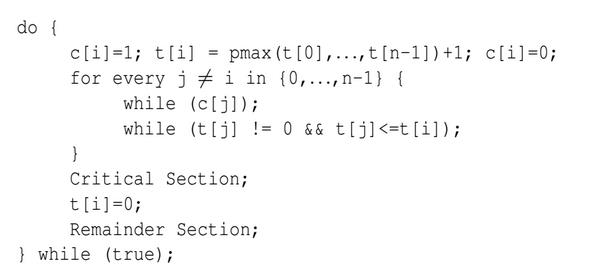Which one of the following is TRUE about the above solution?

Solution:

Mutual exclusion  is satisfied:
All other processes j started before i must have value (i.e. t[j]) less than the value of process i (i.e. t[i])  as function pMax() return a integer not smaller  than any of its arguments. So if anyone out of the processes j have positive value will be executing in its critical section as long as the condition t[j] > 0 && t[j] <= t[i] within while will persist. And when  this j process comes out of its critical section, it sets t[j] = 0;  and next process will be selected in for loop.
So when i process reaches to its critical section none of the  processes j which started earlier before process i  is in its critical section. This ensure that only one process is executing its critical section at a time.

Deadlock and progress are  not satisfied:
while (t[j] != 0 && t[j] <=t[i]); because of this condition deadlock is possible when value of j process becomes equals to the value of process i (i.e t[j] == t[i]).  because of the deadlock progess is also not possible (i.e. Progess == no deadlock) as no one process is able to make progress by stoping other process.

Bounded waiting is also not satisfied:
In this case both deadlock and bounded waiting to be arising from the same reason as if t[j] == t[i] is possible then starvation is possible means infinite waiting.

QUESTION: 13

A counting semaphore was initialized to 10. Then 6 P (wait) operations and 4 V (signal) operations were completed on this semaphore. The resulting value of the semaphore is

Solution:

Initially we have semaphore value = 10 Now we have to perform 6 p operation means when we perform one p operation it decreases the semaphore values to one. So after performing 6 p operation we get, semaphore values = 10 - 6 = 4 and now we have to perform 4 v operation means when we perform one V operation it increases the semaphore values to one. So after performing 4 v operation we get, semaphore values = 4 + 4 = 8. Option (B) is correct.

QUESTION: 14

Processes P1 and P2 use critical_flag in the following routine to achieve mutual exclusion. Assume that critical_flag is initialized to FALSE in the main program.
get_exclusive_access ( ) { if (critical _flag == FALSE) { critical_flag = TRUE ; critical_region () ; critical_flag = FALSE; } }

Consider the following statements.
i. It is possible for both P1 and P2 to access critical_region concurrently.
Which of the following holds?

Solution: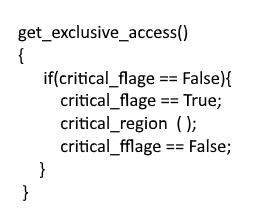Say P1 starts first and executes statement 1, after that system context switches to P2 (before executing statement 2), and it enters inside if statement, since the flag is still false. So now both processes are in critical section!! so (i) is true.. (ii) is false By no way it happens that flag is true and no process' are inside the if clause, if someone enters the critical section, it will definitely make flag = false. So no deadlock.

QUESTION: 15

An operating system contains 3 user processes each requiring 2 units of resource R. The minimum number of units of R such that no deadlocks will ever arise is

Solution:

Total process = 3 and each require 2 units of resource. If we give 1 resource to 1 process then total resource = 1 + 1 + 1 = 3 but in this case deadlock will definitely occur because each process hold 1 unit resource and waiting for another resource so if we increase 1 more resource (3+1=4) then deadlocks will ever arise (i.e., when process 1 complete their execution then it free 2 resource and this 2 resource will used by another process.). Option (B) is correct.